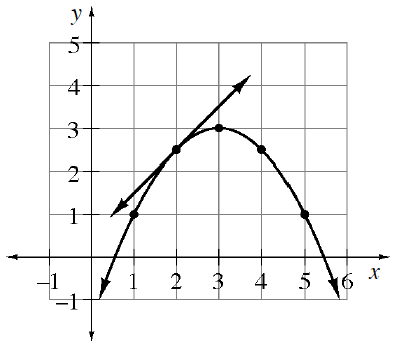### Home > APCALC > Chapter 2 > Lesson 2.4.1 > Problem2-141

2-141.

A tangent line is drawn to the curve $y = -\frac { 1 } { 2 }(x – 3)^2 + 3$ at $x = 2$. Trace the graph on your paper and add tangents at $x = 1, 3, 4,$ and $5$.Use the eTool below to view the tangent lines.
Click on the link to the right to view the full version of the eTool: Slope at a Point eTool